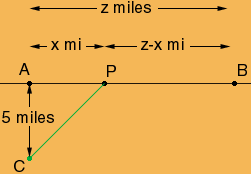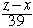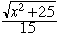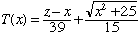A motorist in a desert 5 mi. from point A, which is the nearest point on a long, straight road, wishes to get to point B on the road. If the car can travel 15 mi/hr on the desert and 39 mi/hr on the road to get to B, in the shortest possible time if...... A.) B is 5 mi. from A B.) B is 10 mi. from A C.) B is 1 mi. from A Nancy Secondary student Hi Nancy, In the diagram below the car is at point C. The car is to drive in a straight line to point P on the road and then along the road from P to B. I called the distance from A to P x miles and the distance from A to B z miles. Thus the distance from P to B is z - x miles. In your question z is 5, 10 or 15 miles.Using the Theorem of Pythagoras, the distance from C to P is the square root of x2 + 25 miles. The car travels at 39 mi/hr from P to B and hence takeshours. The car travels at 15 mi/hr from C to P and hence takeshours. Thus the total time, as a function of x is given byYou now have three problems, z = 5, z = 10 and z = 15. For each use your knowledge of calculus to minimize T(x). Cheers, Penny Go to Math Central